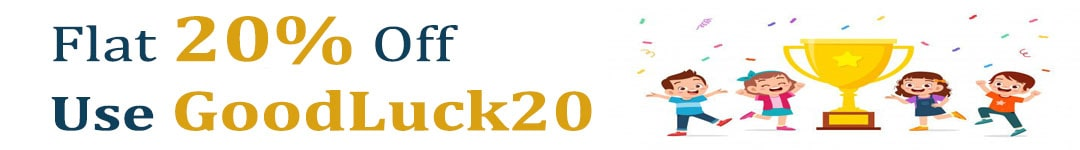International Maths Olympiad Forum By SOF Olympiad Trainer - Page 649

# User ForumSubject :IMO    Class : Class 2

## Ans 1:

Class : Class 10
3622

Subject :IMO    Class : Class 7

## Ans 1:

Class : Class 8
Let tom has Rs. X Let Jasmine has Rs y A.T.Q x+y = 41 x = 41-y 1/4 * x = 2 + 1/7*y 1/4*(41-y) = 2 + 1/7*y 41/4 - y/4 = 2 + y/7 41/4 - 2 = y/7 + y/4 33/4 = 11y/28 33/4*28/11 = y 21 = y x+21=41 X = 41-21 = 20

## Ans 2:

Class : Class 8
Tom and jasmine have Rs 41 altogether . let, Jasmine has Rs x.. therefore tom has Rs( 41-x) {the rest of the money} according to the question, 1/4 of Tom's money is 2 more than 1/7 of jasmine's money. therefore, 1/4 x (41-X) = (1/7 x X)+2 or, ( 41-X)/4=X/7+2 or, (41-X)/4=(X+14)/7 or; (7x41)-7X=4X+(4x14) or; 287-7X=4X+56 or; 287-56=4X+7X or, 231=11X: 11X=231 X=231/11 =21 THE ANSWER IS 21 OR TOM HAS RS 21................

Subject :IMO    Class : Class 7

## Ans 1:

Class : Class 7
80 Rs 5 note and 10 Rs 10 note I have solved by this equation 5x + 10(90-x) = 500

## Ans 2:

Class : Class 8
@ Subarno Maji, that's the correct answer

Subject :IMO    Class : Class 8

## Ans 1:

Class : Class 10
There exist two such numbers : (1-sqrt(5))/2 and (1+sqrt(5))/2 This can be taken out by making a quadratic equation and using the discriminant formula, which is: x=(b*sqrt(b^2-4ac))/2a

Subject :IMO    Class : Class 8

Class : Class 8
115929

## Ans 2:

Class : Class 8
21436587 is exactly divisible by

## Ans 3:

Class : Class 8
99 hehe

Subject :IMO    Class : Class 2

Class : Class 5
90

## Ans 2:

Class : Class 5
90

Subject :IMO    Class : Class 6

#### View All Answers (0)

Subject :IMO    Class : Class 8

## Ans 1:

Class : Class 8
The point where all 3 altitudes of a triangle intersect is called a Orthocenter.

Subject :IMO    Class : Class 8

#### View All Answers (0)

Subject :IMO    Class : Class 8

## Ans 1:

Class : Class 8
yes, it was mentioned in the maths trivia a few days ago. but idk the reason.

## Ans 2:

Class : Class 8
Yes, because of the simple reason, that other polygons have edges! Circle has the same distance from the center at each and every point, whereas the distance from the center to the edges is generally lesser than that to the vertices in other polygons Thus, more area is covered in case of circles.